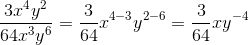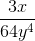# High School Math : Distributing Exponents

## Example Questions

### Example Question #7 : Simplifying Exponents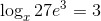Solve for.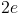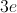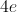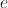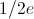Explanation:

First, set up the equation: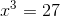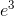. Simplifying this result gives.

### Example Question #1 : Distributing Exponents (Power Rule)

What is the largest positive integer,, such that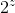is a factor of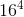?

16

10

5

20

8

16

Explanation: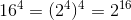. Thus,is equal to 16.

### Example Question #2 : Distributing Exponents (Power Rule)

Order the following from least to greatest: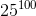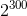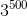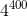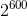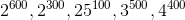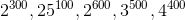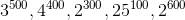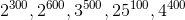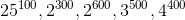Explanation:

In order to solve this problem, each of the answer choices needs to be simplified.

Instead of simplifying completely, make all terms into a form such that they have 100 as the exponent.  Then they can be easily compared.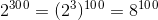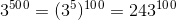,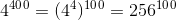, and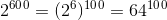.

Thus, ordering from least to greatest: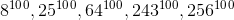.

### Example Question #1 : Integer Exponents

Simplify the expression: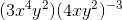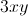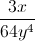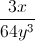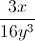Cannot be simplifiedExplanation:

Begin by distributing the exponent through the parentheses. The power rule dictates that an exponent raised to another exponent means that the two exponents are multiplied: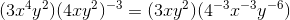Any negative exponents can be converted to positive exponents in the denominator of a fraction: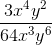The like terms can be simplified by subtracting the power of the denominator from the power of the numerator: